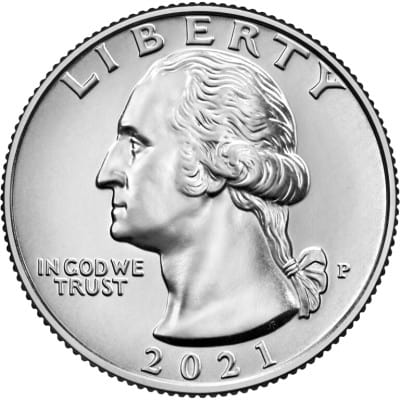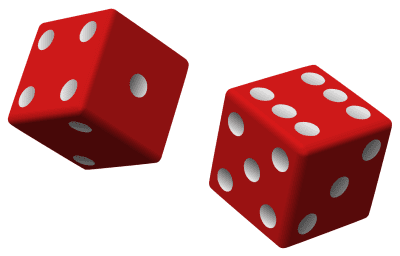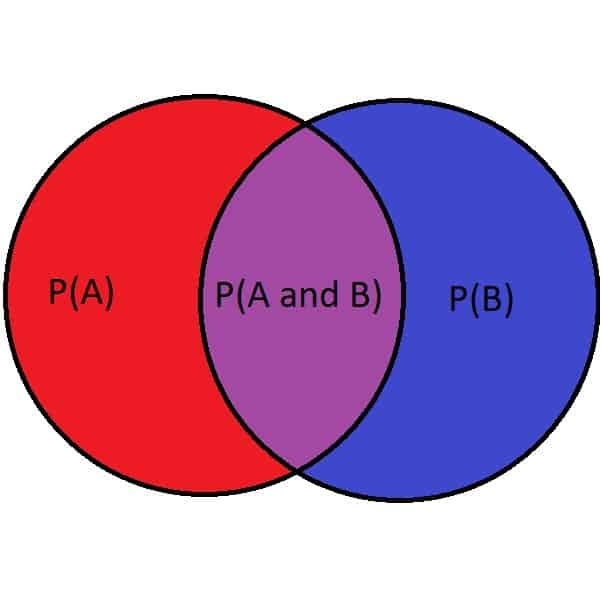# What Is Conditional Probability? (5 Key Ideas To Know)

Conditional probability is a concept that helps us to apply new knowledge when we are making predictions or calculating probabilities.  It relates two events and the probability of one or both happening.

So, what is conditional probability?  The conditional probability P(A|B) is the probability that event A will occur, given that event B has already occurred. Knowing B has already occurred will change the probability that A will occur (unless A & B are independent events). The conditional probability formula is P(A|B) = P(AnB) / P(B).

Of course, we can also find conditional probability from a table if we have enough information.  Certain words (given that, already, etc.) can indicate when we should use conditional probability.

In this article, we’ll talk about what conditional probability is, why we use it, and when to use it.  We’ll also go over some examples with numbers to make the concept clear.

Let’s get started.

## What Is Conditional Probability?

Conditional probability is the likelihood that an event A will occur, assuming that another event B has already occurred.  The idea is this: knowing that event B occurred will sometimes change the probability that A will occur.Conditional probability helps us to use the information we have to refine our probability calculations or estimates. For example, if we know that the temperature is below freezing, it increases the chance of snow.

In symbols, and words: the conditional probability P(A|B) is the probability that event A will occur, given that event B has already occurred.

The conditional probability formula is given by:

• P(A|B) = P(AnB) / P(B)

where:

• P(B) = the probability that event B occurs
• P(AnB) = the probability that event A and B both occur
• P(A|B) = the probability of A given B

For example, let’s say that A is the event that the temperature reaches 70 degrees Fahrenheit today, and B is the event that the temperature reaches 70 degrees Fahrenheit by 10am today.

The probability of event A might be 60%, based on historical data for this day in past years.  However, if event B has already occurred (the temperature is 70 degrees Fahrenheit at 10am), then event A has already occurred (the conditional probability is 100%).If the temperature is already 70 degrees Fahrenheit by 10am, then the high temperature for the day is at least 70 degrees Fahrenheit, regardless of what happens for the rest of the day.

Note that the conditional probability formula for B given A, P(B|A), looks a little different:

• P(B|A) = P(AnB) / P(A)

### Conditional Probability With Independent Events

The formulas above are for conditional probability when events are dependent.  The equation changes to a simpler form when A and B are independent events.

Remember that A and B are independent events if the probability of event B is the same whether A occurs or not, and the probability of event A is the same whether B occurs or not.A coin landing on heads (event A) and a thunderstorm (event B) are independent events, since the occurrence of one does not affect the occurrence of the other.

The equation for this is:

• P(AnB)=P(A)P(B)

If we substitute this equation into the conditional probability formula, we get:

• P(A|B) = P(AnB) / P(B)
• P(A|B) = P(A)P(B) / P(B)
• P(A|B) = P(A)

So, the probability of event A does not change if event B happens.  Likewise, for the conditional probability P(B|A):

• P(B|A) = P(AnB) / P(A)
• P(B|A) = P(A)P(B) / P(A)
• P(B|A) = P(B)

### Conditional Probability Of Mutually Exclusive Events

The formulas for conditional probability change again when A and Be are mutually exclusive events.Rolling a 2 on two dice (event A) and rolling a 12 on two dice (event B) are mutually exclusive, since they cannot both occur at the same time.

Remember that A and B are mutually exclusive events if they cannot both occur at the same time.  The equation for this is given by:

• P(AnB) = 0

That is, the probability of event A and event B happening simultaneously is zero. (Note: for two mutually exclusive events, it is possible that neither one happens).

If we substitute this equation into the conditional probability formula, we get:

• P(A|B) = P(AnB) / P(B)
• P(A|B) = 0 / P(B)
• P(A|B) = 0

So, the conditional probability of event A given event B is zero.  That is, if event B has already happened, then event A cannot happen.

Likewise, for the conditional probability P(B|A):

• P(B|A) = P(AnB) / P(A)
• P(B|A) = 0 / P(A)
• P(B|A) = 0

### Why Do We Need Conditional Probability?

We need conditional probability because not all events are independent.  So, when one event occurs, it tells us something about the chance that the other event occurs.

Without conditional probability, we could not update our numbers to reflect the new information.

It also helps us to run hypothetical scenarios (if we assume event B were to happen, what is the chance that A also happens?)

### When To Use Conditional Probability

Conditional probability is used when we have two events and one has already occurred (or when we are assuming that one has already occurred).What is the probability that a house in Boston will sell for at least \$900,000, given that it has 4 bedrooms and 2 bathrooms? If we have enough information, we can answer this questions with conditional probability.

Here are some of the key words and phrases to look out for that indicate conditional probability:

• “given that” (e.g. the probability of event A given that event B happens).
• “already” (e.g. the probability of event A if B has already occurred)
• “also”  (e.g. if event B occurs, what is the probability that event A also occurs)

### Does Order Matter In Conditional Probability?

Order matters in conditional probability.  That is, P(A|B) and P(B|A) are not necessarily the same number (sometimes they are by chance).

When we write P(A|B), the probability that event A occurs given that event B occurs:

• The first event, A, is the one that may occur (we want to find the probability that it happens)
• The second event, B, is the one that we assume has already occurred (this may affect that chance that A occurs)

We can use the conditional probability formula to see that order matters in conditional probability.

We know that:

• P(A|B) = P(AnB) / P(B)
• P(B|A) = P(AnB) / P(A)

The formulas look similar, but the denominators on the right sides of the equations are different.  The only way that P(A|B) is equal to P(B|A) is when:

• P(A|B) = P(B|A)
• P(AnB) / P(B) = P(AnB) / P(A)
• 1 / P(B) = 1/P(A)
• P(A) = P(B)

So, P(A|B) = P(B|A) if and only if P(A) = P(B).

### How To Calculate Conditional Probability

To calculate conditional probability, we need two other probabilities: P(B) and P(AnB).  If we don’t have them to start with, we may need to calculate them from equations, in context, or with a table.

Let’s look at some examples of how to find conditional probability.

#### Example 1: How To Calculate Conditional Probability

Let’s say that we want to calculate the conditional probability P(A|B).  We know that P(B) = 0.4 and P(AnB) = 0.1.

Using the conditional probability formula, we get:

• P(A|B) = P(AnB) / P(B)
• P(A|B) = 0.1 / 0.4
• P(A|B) = 0.25

So, the probability of A, given that B has already occurred, is 0.25 (a 25% chance).

#### Example 2: How To Calculate Conditional Probability

Let’s say that we want to calculate the conditional probability P(A|B).  We know that P(A) = 0.75, P(B) = 0.6, and P(B|A) = 0.2.

We will need P(AnB) to find P(A|B), and we can find it with the conditional probability formula for P(B|A):

• P(B|A) = P(AnB) / P(A)
• 0.2 = P(AnB) / 0.75
• (0.2)(0.75) = P(AnB)
• 0.15 = P(AnB)

Now, using the conditional probability formula for P(A|B), we get:

• P(A|B) = P(AnB) / P(B)
• P(A|B) = 0.15 / 0.6
• P(A|B) = 0.25

So, the probability of A, given that B has already occurred, is 0.25 (a 25% chance).

#### Example 3: How To Calculate Conditional Probability

Let’s say that we want to calculate the conditional probability P(A|B).  We know that P(A) = 0.3, P(B) = 0.4, and P(AuB) = 0.5.

Remember that P(AuB) (the probability of A or B or both occurring) is different from P(AnB) (the probability that A and B both occur).

The formula to relate P(AuB) and P(AnB) is given by:

• P(AuB) = P(A) + P(B) – P(AnB)

Using the information we were given, we find:

• 0.5 = 0.3 + 0.4 – P(AnB)
• 0.5 = 0.7 – P(AnB)
• -0.2 = – P(AnB)
• 0.2 = P(AnB)

Now, using the conditional probability formula for P(A|B), we get:

• P(A|B) = P(AnB) / P(B)
• P(A|B) = 0.2 / 0.4
• P(A|B) = 0.5

So, the probability of A, given that B has already occurred, is 0.5 (a 50% chance).

#### Example 4: How To Calculate Conditional Probability From A Table

Let’s say that we have the following table of values from a high school:

What is the probability that a student plays baseball, given that he is a junior?We can use conditional probability to find the probability that a student plays baseball, given that he is a junior.

Assign the following events:

• A = the event that the student plays baseball
• B = the event that the student is a junior

Then we want to calculate P(A|B).  That means that we need P(AnB) and P(B).

To find P(AnB), we look for the table entry where “junior” and “baseball” intersect, then divide by the total number of students.

So P(AnB) = 12/200 = 0.06.

To find P(B), we find the total number of juniors, then divide by the total number of students.

So, P(B) = 60/200 = 0.3

Now, using the conditional probability formula for P(A|B), we get:

• P(A|B) = P(AnB) / P(B)
• P(A|B) = 0.06 / 0.3
• P(A|B) = 0.2

So, the probability of A, given that B has already occurred, is 0.2 (a 20% chance).  This means that there is a 20% chance that a student plays baseball, given that he is a junior.

### Can Conditional Probability Be Negative?

Conditional probability cannot be negative.  Any probability always has a value between 0 (0%) and 1 (100%).

A probability of 0 means that the event is guaranteed not to occur, while a probability of 1 means the event is guaranteed to occur.

### Can Conditional Probability Be Greater Than 1?

Conditional probability cannot be greater than 1.  Any probability always has a value between 0 (0%) and 1 (100%).

There is no probability beyond “guaranteed” (100% chance), so a probability cannot be greater than 1.

We can also see why this is true by using the conditional probability formula.  If P(A|B) > 1, then:

• P(A|B) > 1
• P(AnB) / P(B) > 1
• P(AnB) > P(B)

This cannot be true, since AnB (events A and B both occur) is always a more restrictive condition than B (event B occurs).  We can see this from the Venn diagram below:The probability of event B is always at least as large as the probability that events A and B both occur.

### Can Conditional Probability Be Greater Than Unconditional Probability?

Conditional probability can be greater than unconditional probability in some cases.  This happens when knowing that event B has occurred makes it more likely that event A will occur.

For example, let’s say that P(A) = 0.2 (the unconditional probability that event A occurs is 20%).  If P(AnB) = 0.6 and P(B) = 0.8, then the conditional probability formula for P(A|B) gives us:

• P(A|B) = P(AnB) / P(B)
• P(A|B) = 0.6 / 0.8
• P(A|B) = 0.75

So, P(A|B) = 0.75 > 0.2 = P(A).  In this case, the conditional probability is greater than the unconditional probability.

## Conclusion

Now you know what conditional probability is and when to use it.  You also know how to do some of the calculations involved in conditional probability.